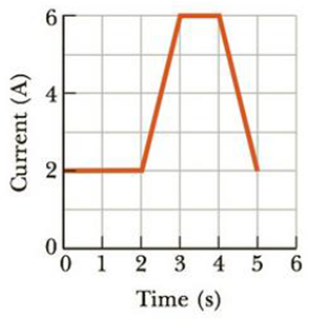Chapter 17, Problem 60AP

Chapter
Section
Textbook Problem

The current in a conductor varies in time as shown in Figure P17.60. (a) How many coulombs of charge pass through a cross section of the conductor in the interval from t = 0 to t = 5.0 s? (b) What constant current would transport the same total charge during the 5.0-s interval as does the actual current?Figure P17.60

(a)

To determine
The charge pass through a cross section of the conductor in the interval from t=0s to t=5.0s .

Explanation

Given Info:

Explanation:

The graph for current in conductor with time,

In the time interval 0st5.0s , the charge passing through the conductor will be the total area under the graph in the current verses time graph of the conductor.

The total area under the graph above will be the sum of the two rectangles and two triangles A, B, C and D respectively.

Thus,

Formula to calculate the total area under the graph is,

• AA is the area of the rectangle A
• AB is the area of the rectangle B
• AC is the area of the triangle C
• AD is the area of the triangle D

Re-write the above equation,

Atot=lAbA+lBbB+12bChC+12bDhD

• lA is the length of the rectangle A
• bA is breadth of the rectangle A
• lB is the length of the rectangle B
• bB is breadth of the rectangle B
• bC is the base of the triangle C
• hC is the height of the triangle C
• bD is the base of the triangle D
• hD is the height of the triangle D

Substitute 5

(b)

To determine
The constant current that transport the same charge during 5.0s interval.

Still sussing out bartleby?

Check out a sample textbook solution.

See a sample solution

The Solution to Your Study Problems

Bartleby provides explanations to thousands of textbook problems written by our experts, many with advanced degrees!

Get Started